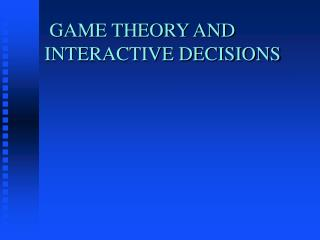DownloadDownload PresentationGAME THEORY AND INTERACTIVE DECISIONS

# GAME THEORY AND INTERACTIVE DECISIONS

Télécharger la présentation## GAME THEORY AND INTERACTIVE DECISIONS

- - - - - - - - - - - - - - - - - - - - - - - - - - - E N D - - - - - - - - - - - - - - - - - - - - - - - - - - -
##### Presentation Transcript

1. GAME THEORY AND INTERACTIVE DECISIONS

2. Introduction • Interactive and Non- Interactive Decisions • Fundamentals of Game Theory • Types of Games • Game Solution and Equilibrium • Non Zero and Zero Sum Games • Summary

3. Fundamentals • Decision Theory: Non Interactive and Interactive Decisions • History of Game Theory • Game Notations in 2 Person Games. • Strategies • Payoffs • Types of Games: Non Zero and Zero Sum Games

4. Games Solutions Concepts • Inferior and dominant strategies, inadmissibility • Dominant Equilibrium • Non zero Sum Games: Competition and Cooperation • Nash Equilibrium • The Prisoner’s Dilemma

5. Zero Sum Games • Solution with Pure Strategies: The Saddle Point Solution • Solution with Mixed Strategies • Strategy graphs and Game Value • Summary of Game Theory